Disclosure: This post may contain affiliate links, meaning when you click the links and make a purchase, we receive a commission.

# How to Compare Two Columns in Excel Using VLOOKUP

There are a lot of ways to compare two lists or columns in Excel. In this article, I am going to compare two columns in excel using VLOOKUP. In one of my previous articles, I discussed How to Compare Two Columns or Lists in Excel where I discussed other methods of comparison.

Before starting the comparison, I will discuss the syntax, arguments and other necessary things about the VLOOKUP function.

## VLOOKUP Function`s Description

The V in VLOOUP stands for “vertical”. VLOOKUP. The VLOOKUP is a built-in function of Excel which performs the vertical lookup by searching a specific value of a column in another column.

The syntax of VLOOKUP function is VLOOKUP( lookup_value, table_array, col_index_number, [range_lookup] ). The arguments which are stated in this syntax have specific meaning.

• Lookup_value– The value which will be used as lookup value for from the 1st
• Table_array– Range of data where the value will be searched.
• Col_index_number-The column from the range from which we will get the value.
• Range_lookup-It is an optional argument. TRUE is used for the approximate match and FALSE is used for the exact match. If this argument is omitted Excel use the TRUE parameter as default.

The results from the VLOOKUP function can be text strings or numeric data depending on the data you are using. If FALSE is used as [range_lookup] then it will find an exact match. If no exact match is found then it will return the value #N/A. If TRUE is used as [range_lookup] then it will look for an approximate match. If no approximate match is found then it will return the next smaller value.

Read More: How to Compare Two Columns in Excel For Finding Differences

## How to Compare Two columns in Excel Using VLOOKUP

Let`s say you have two columns where some colors are listed. I am going to compare these two columns for exact matches. Like, If I choose the color Blue from the 1st column and look up for this color in the 2nd column it will start looking up for the blue color and if this color doesn’t exist then it will return the value #N/A. So, let`s start the comparison.

• The color lists are listed below and in the Existence column, the comparison will be shown.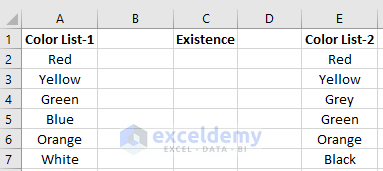• IN cell C2 write the formula  and press enter.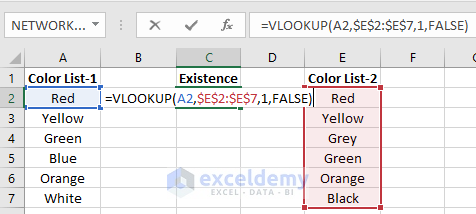• You will see the value red is found in that cell.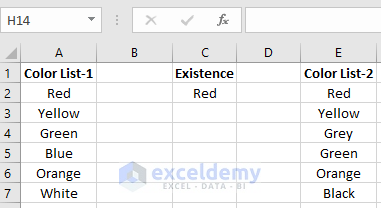• Now drag down the formulated cell to copy the formula for the rest of the cells in the column. You will see the result that you were looking for.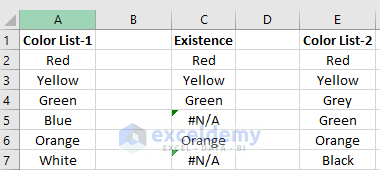• The #N/A results are found because the color Blue and White are not in the Color List-2.

Note: We specify the range as \$E\$2:\$E\$7. The “\$” is used to make the cells absolute and constant in a formula. So, whenever you copy the formula for other cells it will use the same range.

Read More: How to Insert or Delete Rows and Columns from Excel Table

## Using IF, ISNA and VLOOKUP for Comparison Between Two Columns

Here I will be using the same example. But I will insert two new functions with the VLOOKUP function. If there is no exact match between the columns the formula will return NO. If matches exist the formula will return YES with respect to the first column.

• The color lists are listed below and, in the Color Exists column, the comparison will be shown.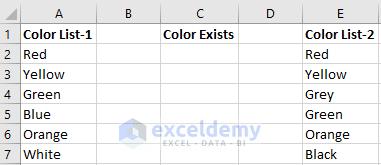• Now write the formula in cell C2 and press enter.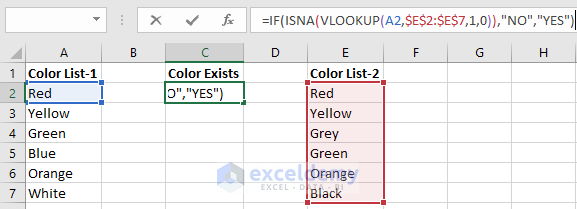• The formula will return the value YES as the color Red exist in the two columns.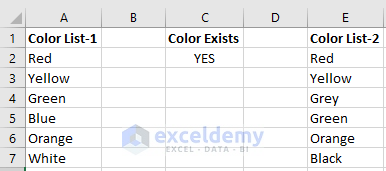• Now drag this formula to the rest of the cell to show the comparison between the two columns.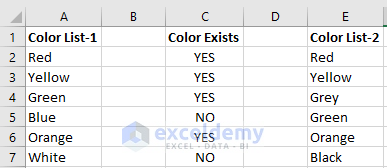• Here we are getting the result NO as the colors Blue and White don’t exist in Color List-2.

Read More:How to Use the HLOOKUP Function in Excel

## How to Compare Two Excel Sheets Using VLOOKUP

In this example, we will compare two columns of two different Excel sheets using VLOOKUP. The formula will be similar except in the Table_array argument there will be an addition of the worksheet name. Let`s say we have two lists of colors in two different worksheets. The worksheets name is defined as CL-1 and CL-2. We will compare the Color List-1 of CL-1 worksheet with Color List 2 of the CL-2 worksheet. The two list form the two worksheets are given below.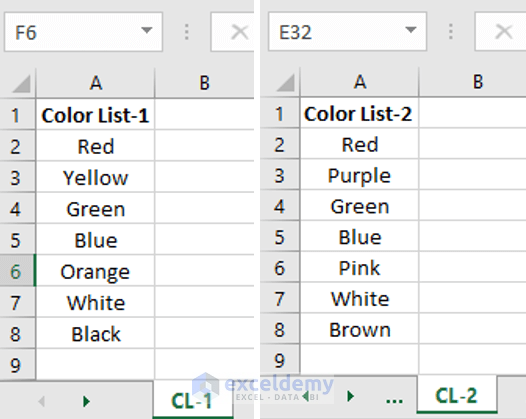• In cell B2 of CL-1 worksheet write the formula and press enter.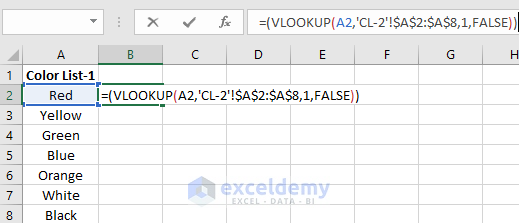• In cell B2 you will see the value Red appears. This is because the color Red is common color in both the columns of CL-1 and CL-2 worksheets.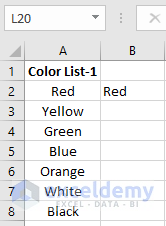• Now copy this formula from cell B3 to B8 to find the result for the whole two columns.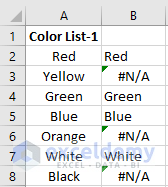Here the color Yellow, Orange, and Black don’t appear in CL-2 worksheet. That’s why we get the rest #N/A in CL-1 worksheet.

## Conclusion

It`s important to know the VLOOKUP function properly as any small mistake in the arguments will not give you the result you wanted. You can use the MATCH and INDEX functions as an alternative to the VLOOKUP functions.

How to Highlight Every Other Row in Excel

How to Delete Blank Rows in Excel (6 Ways)

How to Lock and Unlock Certain/Specific Cells in Excel

How to Multiply Columns, Cells, Rows, & Numbers in Excel

How to Find Duplicate Values in Excel using VLOOKUP

Hello!

Welcome to my Profile. Here I will be posting articles related to Microsoft Excel. I have completed my BSc in Electrical and Electronic Engineering from American International University-Bangladesh. I am a diligent, goal-oriented engineer with an immense thirst for knowledge and attitude to grow continuously. Continuous improvement and life-long learning is my motto.

1 Comment
1. ReplyApril 2, 2019 at 3:00 PM

Thanks for sharing this guide on Combining Excel Files. I have a Suggestion we can Also use Synkronizer Excel Add-in to Combine and Merge Multiple Excel Files into one file.